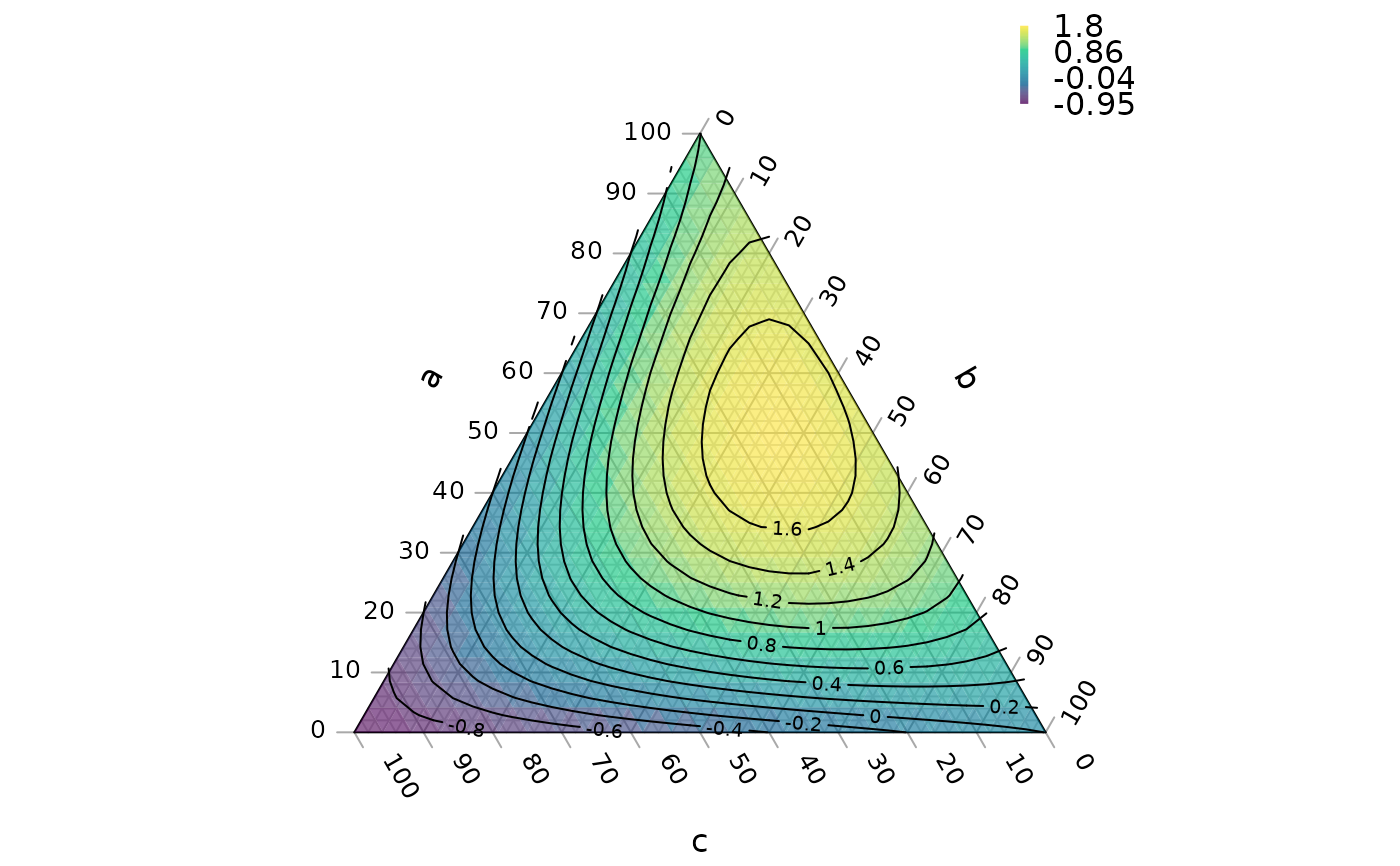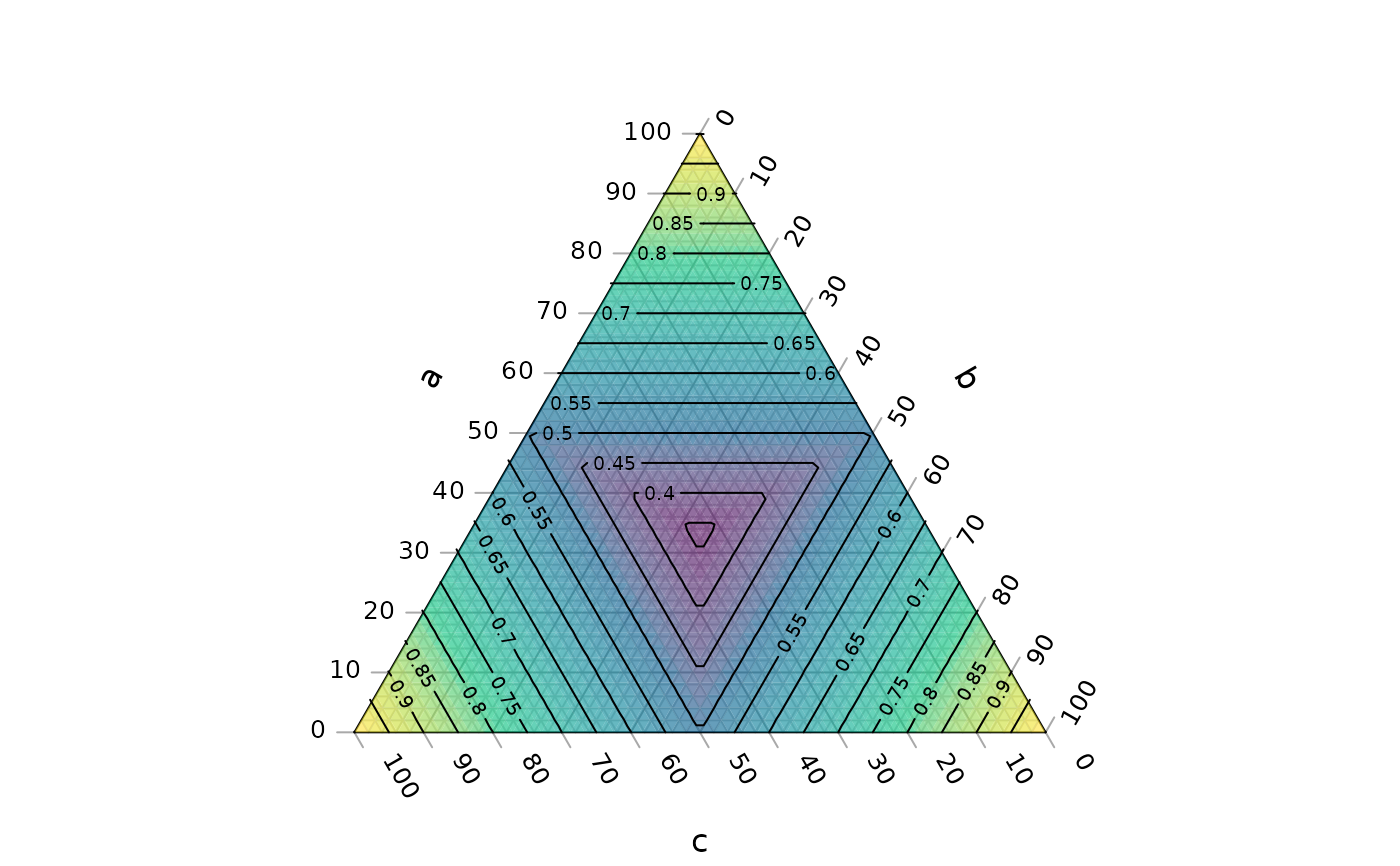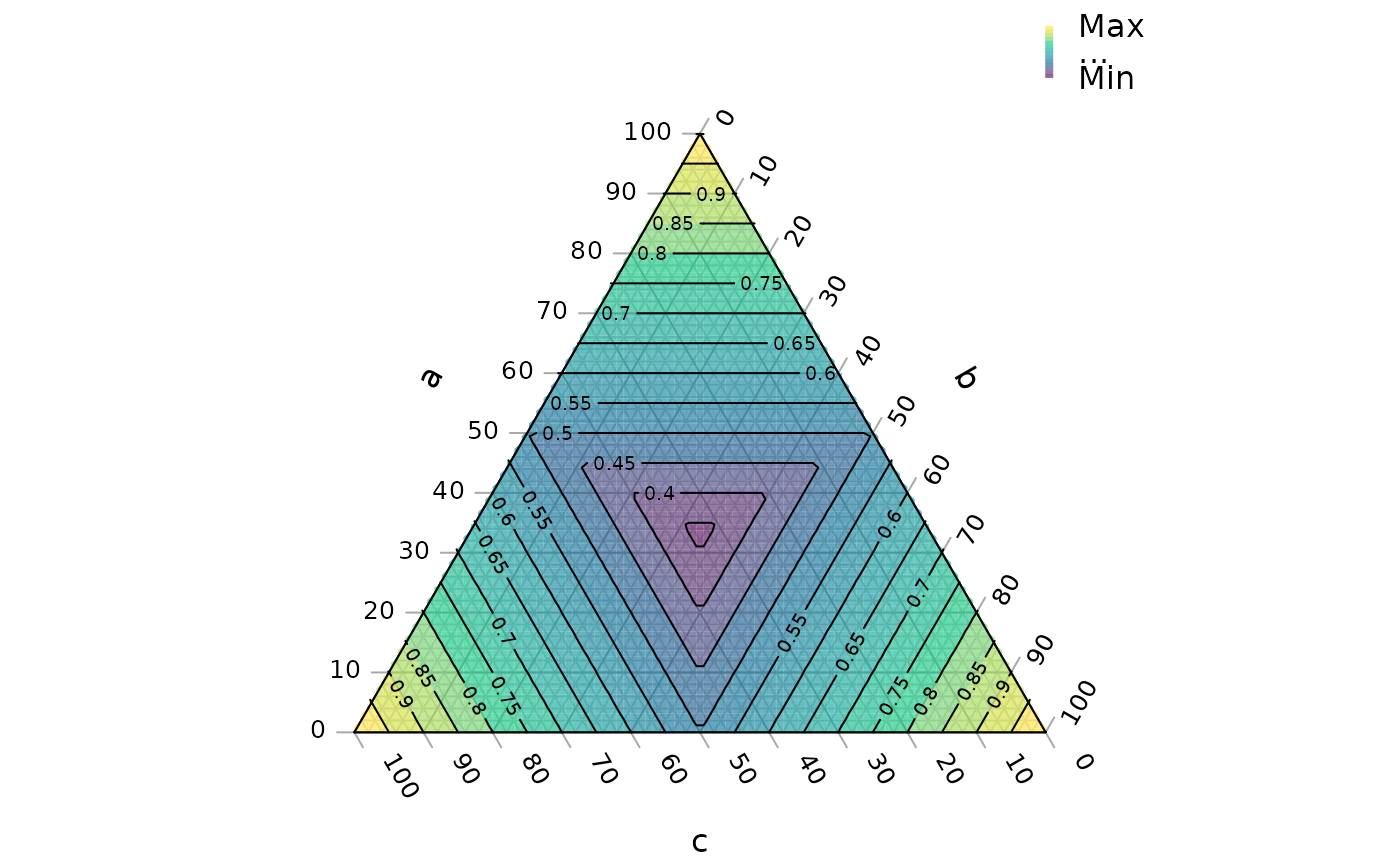Draws contour lines to depict the value of a function in ternary space.

TernaryContour(
Func,
resolution = 96L,
direction = getOption("ternDirection", 1L),
within = NULL,
...
)

## Arguments

Func

Function taking vectors of coordinates a, b and c, which returns a numeric vector whose value at each coordinate will be depicted.

resolution

The number of triangles whose base should lie on the longest axis of the triangle. Higher numbers will result in smaller subdivisions and smoother colour gradients, but at a computational cost.

direction

(optional) Integer specifying the direction that the current ternary plot should point: 1, up; 2, right; 3, down; 4, left.

within

List or matrix of x, y coordinates within which contours should be evaluated, in any format supported by xy.coords(x = within). If NULL, defaults to a region slightly smaller than the ternary plot. The \$hull entry generated by TriangleInHull() may also be used.

...

Further parameters to pass to contour().

Other contour plotting functions: ColourTernary(), TernaryDensityContour(), TernaryPointValues()

## Examples

TernaryPlot(alab = "a", blab = "b", clab = "c")

FunctionToContour <- function (a, b, c) {
a - c + (4 * a * b) + (27 * a * b * c)
}

values <- TernaryPointValues(FunctionToContour, resolution = 24L)
ColourTernary(values)
TernaryContour(FunctionToContour, resolution = 36L)# Note that FunctionToContour is sent a vector.
BadMax <- function (a, b, c) {
max(a, b, c)
}

# Use
GoodMax <- function (a, b, c) {
pmax(a, b, c)
}
TernaryPlot(alab = "a", blab = "b", clab = "c")
ColourTernary(TernaryPointValues(GoodMax))
TernaryContour(GoodMax)# Or, for a generalizable example,
GeneralMax <- function (a, b, c) {
apply(rbind(a, b, c), 2, max)
}
TernaryPlot(alab = "a", blab = "b", clab = "c")
ColourTernary(TernaryPointValues(GeneralMax))
TernaryContour(GeneralMax)